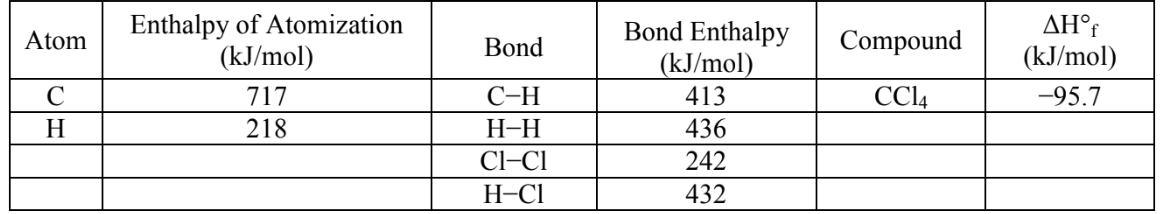# Problem: Estimate the enthalpy of reaction, ΔH rxn°, for each of the following reactions using the information provided. Show all work and circle your final answers.C (s) + 2 H2 (g) → CH4 (g)              ΔH°rxn = ?a. -312 kJb. -221 kJc. -72 kJd. +63 kJe. +740 kJ

###### FREE Expert Solution

For this problem, we have to calculate the enthalpy of reaction (ΔH) using the given bond enthalpies.

C (s) + 2 H2 (g) → CH4 (g)

Recall that the change of enthalpy for a chemical reaction (ΔH) can be calculated using bond energies:

To determine the enthalpy of reaction, we need to first see what bonds are present in the reactants and the products.

79% (230 ratings)###### Problem Details

Estimate the enthalpy of reaction, ΔH rxn°, for each of the following reactions using the information provided. Show all work and circle your final answers.C (s) + 2 H2 (g) → CH4 (g)              ΔH°rxn = ?

a. -312 kJ

b. -221 kJ

c. -72 kJ

d. +63 kJ

e. +740 kJ